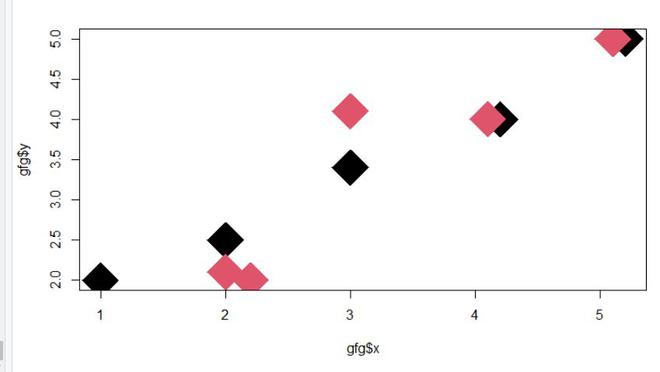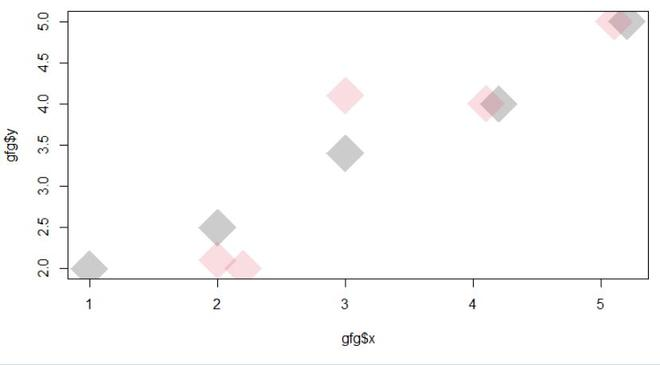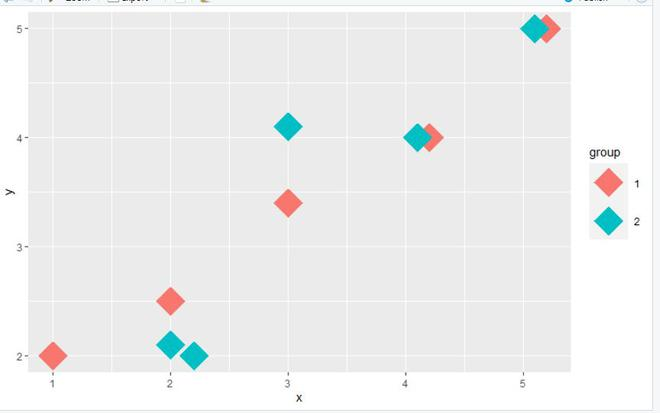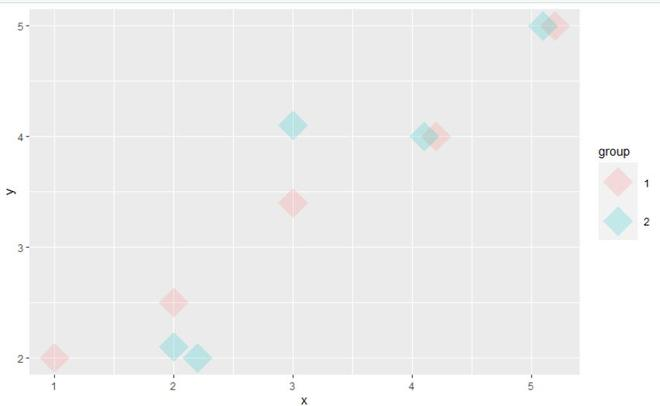# Transparent Scatterplot Points in Base R and ggplot2

• Last Updated : 14 Sep, 2021

In this article, we are going to see how to make transparent scatterplot points in the R programming language.

Here we will use alpha parameter inside the plot. It is used to modify color transparency, alpha value=1 is by default and if we make the alpha value nearer to zero it will be making the object more transparent and on the other hand, the alpha value nearer to 1 will leading to make the object opaque.

## Method 1: Using Base R Programming

In this approach to make transparent scatterplot points, the user needs to install and import the scales package in the working console of the R and this package here is responsible to adjust the alpha of given data points. Further, the user needs to simply call the plot function with the additional alpha argument with this function and specify the alpha value accordingly as per the requirements to make transparent scatterplot points in the base R programming language.

To install and import the scales package, the user needs to follow the below syntax:

```install.packages("scales")
library("scales")  ```

Example: In this example, we will be plotting an of the given data and will be setting the value of the alpha argument to 0.2 to transparent effect in the plotted scatterplot in the R programming language.

The plot without any transparent effect looks as below:

## R

 `library``(``"scales"``)  ` ` `  `gfg <- ``data.frame``(x = ``c``(1, 2.2, 2, 2, 3, 3, ` `                        ``4.2, 4.1, 5.2, 5.1),   ` `                   ``y = ``c``(2, 2, 2.5, 2.1, 3.4, ` `                         ``4.1, 4, 4, 5, 5), ` `                ``group = ``as.factor``(1:2)) ` ` `  `plot``(gfg\$x, gfg\$y, pch = 18, cex = 6, ` `     ``col = gfg\$group)`

Output:Using alpha to create a transparent plot:

## R

 `library``(``"scales"``)  ` ` `  `gfg <- ``data.frame``(x = ``c``(1, 2.2, 2, 2, 3, 3, ` `                        ``4.2, 4.1, 5.2, 5.1),   ` `                   ``y = ``c``(2, 2, 2.5, 2.1, 3.4, ` `                         ``4.1, 4, 4, 5, 5), ` `                ``group = ``as.factor``(1:2)) ` ` `  `plot``(gfg\$x,gfg\$y, pch = 18, cex = 6, ` `     ``col = ``alpha``(gfg\$group, 0.2))`

Output:## Method 2: Using ggplot2 Package

Transparent scatterplot points using the alpha argument of the geom_point()  function, in this approach to make transparent scatterplot points, the user needs to install and import the ggplot2 package in the working console of the R and this package here is responsible to plot the ggplot2 scatter plot of given data points. Further, the user needs to call the geom_point()  function of the ggplot2 package with the additional alpha argument with this function and specify the alpha value accordingly as per the requirements to make transparent scatterplot points in the gglot2 R programming language.

To install and import the scales package, the user needs to follow the below syntax:

```install.packages("ggplot2")
library("ggplot2") ```

Example: In this example, we will be plotting an of the given data and will be setting the value of the alpha argument to 0.2 to transparent effect in the plotted scatterplot of the ggplot package in the R programming language.

The plot without any transparent effect looks as below:

## R

 `library``(``"ggplot2"``)  ` ` `  `gfg <- ``data.frame``(x = ``c``(1, 2.2, 2, 2, 3, 3, ` `                        ``4.2, 4.1, 5.2, 5.1),   ` `                   ``y = ``c``(2, 2, 2.5, 2.1, 3.4, ` `                         ``4.1, 4, 4, 5, 5), ` `                ``group = ``as.factor``(1:2)) ` ` `  `ggplot``(gfg, ``aes``(x, y, col = group)) +  ` `geom_point``(pch = 18,size = 12)`

Output:Using alpha to create a transparent plot:

## R

 `library``(``"ggplot2"``)  ` ` `  `gfg <- ``data.frame``(x = ``c``(1, 2.2, 2, 2, 3, 3, 4.2, ` `                        ``4.1, 5.2, 5.1),   ` `                   ``y = ``c``(2, 2, 2.5, 2.1, 3.4, 4.1, ` `                         ``4, 4, 5, 5), ` `                ``group = ``as.factor``(1:2)) ` `ggplot``(gfg, ``aes``(x, y, col = group)) +  ` `geom_point``(pch = 18,size = 12, alpha = 0.2)`

Output:My Personal Notes arrow_drop_up
Recommended Articles
Page :